# 9 Classification Methods From Spark MLlib We Should KnowHow about a classifier to classify humans characters into Good, Bad or Ugly! Image source.

Spark MLlib is a distributed machine learning framework comprising a set of popular machine learning libraries and utilities. As this use Spark Core for parallel computing, so really useful to apply the algorithms on big data sets.

In this blog, we’ll use 9 well known classifiers to classify the Banknote dataset (download from here and for details, refer here). Instead of going deep into the algorithms or mathematical details, we have limited our discussion on using the Spark MLlib classification methods only. We haven’t included further model optimization/hyper-parameter tuning which can be part of further detailed discussions.

The database contains the following details:

`1. Variance of Wavelet Transformed image2. Skewness of Wavelet Transformed image3. Kurtosis of Wavelet Transformed image4. Entropy of image5. Class variable (0 or 1)`

Let’s load the data and use it as a Spark table…

`df = spark.table ('data_banknote_authentication')print(f"""There are {df.count()} records in the dataset.""")labelCol = "Class"df.show(5)`

# Data Analysis

If we want to have a quick look about how the data are distributed around the mean, we can describe the dataset:

If we want to see any opportunity of reducing dimensions, we can plot the scatter matrix.

Now, let’s see a quick definition of 3 main components of MLlib:

# Estimator, Transformer & Pipeline

Estimator: An Estimator is an algorithm that fits or trains on data. This implements a fit() method, which accepts a Spark DataFrame and produces a Model. E.g. pyspark.ml.classification.LogisticRegression is an estimator.

Transformer: A Transformer is an abstraction that includes feature transformers and learned models. This implements transform() method which transforms one DataFrame into another DataFrame. E.g., an ML model is a Transformer which transforms a DataFrame with features into a DataFrame with predictions.

Pipeline: A Pipeline chains multiple Transformers and Estimators together to specify an ML workflow. This not mandatory to use but this really helps to connect the dots i.e. stages in appropriate order.

# Data Preprocessing

In the dataset, the last attribute is the dependent variable/label and rest are independent attributes or features. Let’s extract the features & labels:

`trainDF, testDF = df.randomSplit([.8, .2], seed=42)print(f"""There are {trainDF.count()} rows in the training set, and {testDF.count()} in the test set""")`

VectorAssembler is a transformer that combines a given list of columns into a single vector column. It is useful for combining raw features and features generated by different feature transformers into a single feature vector, in order to train ML models.

`# Here, the last column is the category columninputCols = trainDF.columns[0: len (trainDF.columns) - 1]from pyspark.ml.feature import VectorAssemblervecAssembler = VectorAssembler(\                               inputCols = inputCols, \                               outputCol = "features") \                              .setHandleInvalid("skip")`

As we’re using Pipeline, we don’t need to individually transform the stages e.g. VectorAssembler or StandardScaler. But, just to show how would the features appear after transforming, let’s execute the following:

`# Optional, as we are using PipelinevecTrainDF = vecAssembler.transform(trainDF)vecTrainDF.select ("features").show(5, False)`

Some algorithms need scaling the features into a same scale while some others (e.g. tree based algorithms) are invariant to it. This process is called Feature Scaling. In this blog we’ll use StandardScaler.

`from pyspark.ml.feature import StandardScalerstdScaler = StandardScaler(inputCol="features", \                        outputCol="scaledFeatures", \                        withStd=True, \                        withMean=False)`

The following code block is optional.

`# Optional - as we're using Pipeline# Compute summary statistics by fitting the StandardScalerscalerModel = stdScaler.fit(vecTrainDF)# Normalize each feature to have unit standard deviation.scaledDataDF = scalerModel.transform(vecTrainDF)scaledDataDF.select ("scaledFeatures").show(5, False)`

# 1. Logistic Regression

Logistic Regression is a classification algorithm created based on the logistic function — Sigmoid activation function to convert the outcome into categorical value. This function produces a S-shaped curve which takes any number as input and produces an output in-between 0 and 1 (in case of Binary Logistic Regression).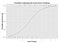Example: Graph of a logistic regression curve showing probability of passing an exam versus hours studying (reference: Wikipedia).Reference: https://www.saedsayad.com/logistic_regression.htm

Logistic regression can be of three types:

1. Binomial / Binary: Dependent variable can have only two possible types, “0” and “1”.
2. Multinomial: Dependent variable can have three or more possible types.
3. Ordinal: Dependent variables that are ordered.

Here, we’ll use only the Binomial one to predict the output.

Training the Logistic Regression model (reference: library) on the training set:

`from pyspark.ml.classification import LogisticRegressionlr = LogisticRegression(maxIter=100, \                        regParam=0.3, \                        elasticNetParam=0.1, \                        featuresCol="scaledFeatures", \                        family = "binomial", \                        labelCol=labelCol)from pyspark.ml import Pipelinepipeline_lr = Pipeline(stages=[vecAssembler, stdScaler, lr])pipelineModel_lr = pipeline_lr.fit(trainDF)`

The parameters used (reference: library):

• maxIter: max number of iterations (>= 0).
• regParam: regularization parameter.
• elasticNetParam: the ElasticNet mixing parameter, in range [0, 1]. For alpha = 0, the penalty is an L2 penalty. For alpha = 1, it is an L1 penalty.
• family: the name of family which is a description of the label distribution to be used in the model; options: auto, binomial, multinomial.

Predicting the test set results:

`predDF_lr = pipelineModel_lr.transform(trainDF)`

Measuring accuracy: we’ll use MulticlassClassificationEvaluator to evaluate the models. This can calculate different metrics such as, f1, accuracy, precisionByLabel etc. Here, we’ll only measure the accuracy.

`from pyspark.ml.evaluation import MulticlassClassificationEvaluatorevaluator = MulticlassClassificationEvaluator( \                  labelCol=labelCol, \                  predictionCol="prediction", \                  metricName="accuracy")`

Once the evaluator is created, we can use it to measure performance of difference models.

`lr_accuracy = evaluator.evaluate(predDF_lr)print("Accuracy of LogisticRegression is = %g"%(lr_accuracy))print("Test Error of LogisticRegression = %g "%(1.0 - lr_accuracy))`

# 2. Linear Support Vector Machine

This model returns a best-fit hyperplane that divides, or categorizes, the input data. This assumes that the data are linearly separable.

# Implementation

Training the SVC model (reference: library) on the training set:

`from pyspark.ml.classification import LinearSVClsvc = LinearSVC(maxIter=10, \                 regParam=0.1, \                 featuresCol="scaledFeatures", \                 labelCol=labelCol)from pyspark.ml import Pipelinepipeline_lsvc = Pipeline(stages=[vecAssembler, stdScaler, lsvc])pipelineModel_lsvc = pipeline_lsvc.fit(trainDF)`

Predicting the test set results:

`predDF_lsvc = pipelineModel_lsvc.transform (testDF)`

Measuring accuracy:

`lr_accuracy = evaluator.evaluate(predDF_lsvc)print("Accuracy of LogisticRegression is = %g"%(lr_accuracy))print("Test Error of LogisticRegression = %g "%(1.0 - lr_accuracy))`

# 3. Naïve Bayes

Naïve Bayes classifiers are a collection of algorithms based on Bayes’ Theorem.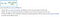Bayes’ Theorem — mathematical formula, source: Wikipedia

All naïve Bayes classifiers assume that the value of a particular feature is independent of the value of the other features, given the class variable. Given, class variable y and dependent feature vector x1 through xn,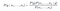Refer the Probabilistic model for further reading.

Few classifiers (in this blog we’ll use Gaussian NB):

• Multinomial (default),
• Bernoulli and
• Gaussian

Training the NB model (reference: library) on the training set:

`from pyspark.ml.classification import NaiveBayesnb = NaiveBayes(smoothing=1.0, \                modelType="gaussian", \                featuresCol="scaledFeatures", \                labelCol=labelCol)from pyspark.ml import Pipelinepipeline_nb = Pipeline(stages=[vecAssembler, stdScaler, nb])pipelineModel_nb = pipeline_nb.fit(trainDF)`

Predicting the test set results:

`predDF_nb = pipelineModel_nb.transform (testDF)`

Measuring accuracy:

`nb_accuracy = evaluator.evaluate(predDF_nb)print("Accuracy of Naïve Bayes is = %g"%(nb_accuracy))print("Error of Naïve Bayes is = %g "%(1.0 - nb_accuracy))`

# 4. Decision Tree

A decision tree is a series of if-then-else rules learned from your data for classification or regression tasks. This method is commonly used in data mining.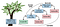Image source: Learning Spark, 2nd Edition, figure 10–9. Decision tree example.

Training the Decision Tree model (reference: library) on the training set:

`from pyspark.ml.classification import DecisionTreeClassifier# Train a DecisionTree model.dt = DecisionTreeClassifier(labelCol=labelCol, \                            featuresCol="scaledFeatures", \                            impurity="gini")from pyspark.ml import Pipelinepipeline_dt = Pipeline(stages=[vecAssembler, stdScaler, dt])pipelineModel_dt = pipeline_nb.fit(trainDF)`

The impurity parameter selects the function to calculate information gain and the information gain is used to split a node:

• gini
• entropy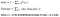Reference: source

Predicting the test set results:

`predDF_dt = pipelineModel_dt.transform (testDF)`

Measuring accuracy:

`dt_accuracy = evaluator.evaluate(predDF_dt)print("Accuracy of Decision Tree is = %g"%(dt_accuracy))print("Error of Decision Tree is = %g "%(1.0 - dt_accuracy))`

# 5. Random Forest

Random Forest is an ensemble of Decision Trees by taking various sub-samples of the dataset and uses averaging or majority voting to improve the predictive accuracy. It’s based on the concept that, averaging/voting predictions from different models will be more robust than a prediction of any individual model.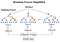Image source: Wikipedia

Training the Random Forest model (reference: library) on the training set:

`from pyspark.ml.classification import RandomForestClassifier# Train a RandomForest model.rf = RandomForestClassifier(labelCol=labelCol, \                            featuresCol="scaledFeatures", \                            numTrees=50)from pyspark.ml import Pipelinepipeline_rf = Pipeline(stages=[vecAssembler, stdScaler, rf])pipelineModel_rf = pipeline_rf.fit(trainDF)`

Few of the parameters (reference library):

• featureSubsetStrategy: The number of features to consider for splits at each tree node. Supported options: ‘auto’ (choose automatically for task: If numTrees == 1, set to ‘all’. If numTrees > 1 (forest), set to ‘sqrt’ for classification and to ‘onethird’ for regression), ‘all’ (use all features), ‘onethird’ (use 1/3 of the features), ‘sqrt’ (use sqrt(number of features)), ‘log2’ (use log2(number of features)), ’n’ (when n is in the range (0, 1.0], use n * number of features. When n is in the range (1, number of features), use n features). default = ‘auto’.
• impurity: Criterion used for information gain calculation (case-insensitive), options: ‘entropy’, ‘gini’.
• maxDepth: Maximum depth of the tree.
• numTrees: Number of trees to train.
`predDF_rf = pipelineModel_rf.transform (testDF)`

Measuring accuracy:

`rf_accuracy = evaluator.evaluate(predDF_rf)print("Accuracy of Random Tree is = %g"%(rf_accuracy))print("Error of Random Tree is = %g "%(1.0 - rf_accuracy))`

# 6. Gradient-Boosted Tree

Gradient-boosted trees (GBTs) are a popular classification and regression method using ensembles of decision trees. These iteratively train decision trees in order to minimize a loss function.

Training the GBT classifier model (reference library) on the training set:

`from pyspark.ml.classification import GBTClassifier# Train a GBT model.gbt = GBTClassifier(labelCol=labelCol, \                    featuresCol="scaledFeatures", \                    maxIter=10)from pyspark.ml import Pipelinepipeline_gbt = Pipeline(stages=[vecAssembler, stdScaler, gbt])pipelineModel_gbt = pipeline_gbt.fit(trainDF)`

Predicting the test set results:

`predDF_gbt = pipelineModel_gbt.transform (testDF)`

Measuring accuracy:

`gbt_accuracy = evaluator.evaluate(predDF_gbt)print("Accuracy of Gradient-Boosted Tree is = %g"%(gbt_accuracy))print("Error of Gradient-Boosted Tree is = %g "%(1.0 - gbt_accuracy))`

# 7. MLP Classifier

Multi-Layer Perceptron (MLP) is a class of artificial neural network (ANN). It has at-least three layers of nodes: an input layer, a hidden layer and an output layer.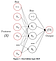Image source: Scikit Learn

Training the MLP classifier model (reference library) on the training set:

`from pyspark.ml.classification import MultilayerPerceptronClassifier# specify layers for the neural network:# input layer of size 4 (features), two intermediate of size 5 and 4# and output of size 2 (classes)layers = [4, 5, 4, 2]# create the trainer and set its parametersmlp = MultilayerPerceptronClassifier(labelCol=labelCol, \                                     featuresCol="scaledFeatures", \                                     maxIter=100, layers=layers, \                                     blockSize=128, \                                     seed=1234)from pyspark.ml import Pipelinepipeline_mlp = Pipeline(stages=[vecAssembler, stdScaler, mlp])pipelineModel_mlp = pipeline_mlp.fit(trainDF)`

Predicting the test set results:

`predDF_mlp = pipelineModel_mlp.transform (testDF)`

Measuring accuracy:

`mlp_accuracy = evaluator.evaluate(predDF_mlp)print("Accuracy of MLP Classifier is = %g"%(mlp_accuracy))print("Error of MLP Classifier is = %g "%(1.0 - mlp_accuracy))`

# 8. One-vs-Rest

OneVsRest is an example of a machine learning reduction for performing multiclass classification given a base classifier that can perform binary classification efficiently. It is also known as “One-vs-All.” — reference.

Training the OvR classifier model (reference library) on the training set:

`from pyspark.ml.classification import OneVsRestovr = OneVsRest(classifier=lr, \                labelCol=labelCol, \                featuresCol="scaledFeatures")from pyspark.ml import Pipelinepipeline_ovr = Pipeline(stages=[vecAssembler, stdScaler, ovr])pipelineModel_ovr = pipeline_ovr.fit(trainDF)`

Predicting the test set results:

`predDF_ovr = pipelineModel_ovr.transform (testDF)`

Measuring accuracy:

`ovr_accuracy = evaluator.evaluate(predDF_ovr)print("Accuracy of One-vs-Rest is = %g"%(ovr_accuracy))print("Error of One-vs-Rest is = %g "%(1.0 - ovr_accuracy))`

# 9. Factorization Machines

Factorization Machines are able to estimate interactions between features even in problems with huge sparsity… The spark.ml implementation supports factorization machines for binary classification and for regression. reference.

Training the FM classifier model (reference library) on the training set:

`from pyspark.ml.classification import FMClassifier# Train a FM model.fm = FMClassifier(labelCol=labelCol, \                  featuresCol="scaledFeatures", \                  stepSize=0.001)from pyspark.ml import Pipelinepipeline_fm = Pipeline(stages=[vecAssembler, stdScaler, fm])pipelineModel_fm = pipeline_fm.fit(trainDF)`

One of the parameters we’re passing here (reference library):

• stepSize: Step size to be used for each iteration of optimization.

Predicting the test set results:

`predDF_fm = pipelineModel_fm.transform (testDF)`

Measuring accuracy:

`fm_accuracy = evaluator.evaluate(predDF_fm)print("Accuracy of Factorization Machines is = %g"%(fm_accuracy))print("Error of Factorization Machines is = %g "%(1.0 - fm_accuracy))`

Further reference:

# Conclusion

In case we need to work with massive amount of data, Spark MLlib is a good choice. Otherwise, for limited data we can try the single-node framework - Scikit Learn.

For using classifiers from Scikit Learn check this out — 10 Classification Methods From Scikit Learn We Should Know.

Thanks for reading!! If you have enjoyed, Clap & Share it!! To see similar posts, follow me on Medium & LinkedIn.

Tech enthusiast, Azure Big Data Architect.

## More from Prosenjit Chakraborty

Tech enthusiast, Azure Big Data Architect.